# Quiz 1: Measurement and Problem Solving

Physics & Astronomy

The physical quantities are introduced for the purpose of measurements. The fundamental physical quantities are the independent physical quantities and all other physical quantities depend on these fundamental quantities. The SI units of fundamental physical quantities are nothing but base units in the SI system. The seven fundamental physical quantities are mass, length, time, current, temperature, amount of substance and intensity of light. The base units in the SI system are: Kilogram, meter, second, ampere, kelvin, mole and candela. Any units other these can be expressed in terms of these units. That is, the base units are enough to express any other units, using different combinations among them. This is why there are not more SI base units.

The metric system is a base-10 (decimal) system and British system is a base-12(duodecimal) system. In the decimal system (base-10), a dime is equal to 10 cents.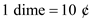Here,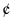is symbol of penny. One dollar is equal to 10 dimes.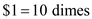Substitute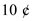for dime in the above equation.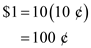Similarly, in a duodecimal (base-12) system, a dime is equal to 12 ¢.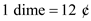A dollar is equal to 12 dimes.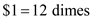Substitutefor dime in the above equation.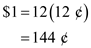Rewrite equation for.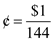Therefore, a penny would be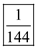of a decimal dollar.

International system of units is the recent description of metric system and it is abbreviated as SI. The SI system consists of both base units and derived units. There are seven base units of SI system. They are as follows: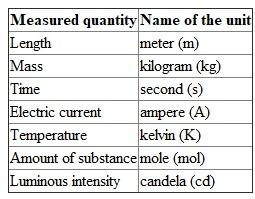Thus, there are seven base units in the SI system. Hence, the correct option is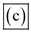.

There is no answer for this question